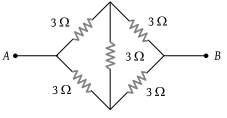NEET Questions Solved

The equivalent resistance of the following diagram A and B is(1) $\frac{2}{3}\Omega$

(2) 9 Ω

(3) 6 Ω

(4) None of these

(4) Equivalent resistance of the given circuit is $3\Omega$.

Difficulty Level:

• 11%
• 9%
• 9%
• 73%